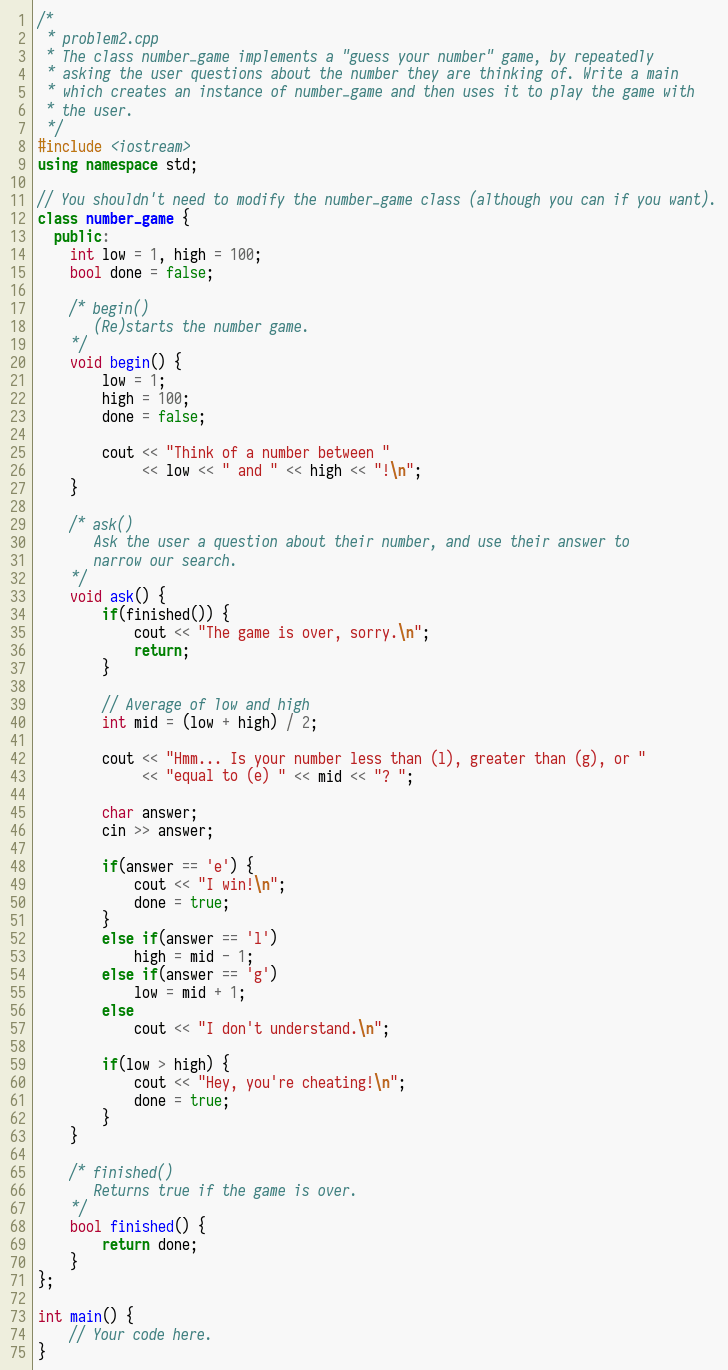# Question: “NONO * Problem2.cpp * The Class Number-game Implements A “”guess Your Number”” Game, By Repeatedly * Asking The User Questions About The Number They Are Thinking Of. Write A Main * Which Creates An Instance Of Number-game And Then Uses It To Play The Game With * The User. #include Using Namespace Std; 11 // You Shouldn’t Need To Modify The Number-game …”

NONO * Problem2.cpp * The Class Number-game Implements A “guess Your
Number” Game, By Repeatedly * Asking The User Questions About The Number
They Are Thinking Of. Write A Main * Which Creates An Instance Of
Number-game And Then Uses It To Play The Game With * The User. #include
Using Namespace Std; 11 // You Shouldn’t Need To Modify The Number-game …

Draw the major product formed in the following reactionNONO * problem2.cpp * The class number-game implements a “guess your number” game, by repeatedly * asking the user questions about the number they are thinking of. Write a main * which creates an instance of number-game and then uses it to play the game with * the user. #include using namespace std; 11 // You shouldn’t need to modify the number-game class (although you can if you want). 12 class number-game { public: int low = 1, high = 100; bool done = false; /* begin() (Re)starts the number game. void begin() { low = 1; high = 100; done = false; cout

Smart Teacher

solution:

given data:

#include<iostream>
using namespace std;
class number_game{
public:
int low=1,high=100;
bool done=false;

void begin()
{
low=1;
high=100;
done=false;

cout<<“Think of a number between “<<low<<” and “<<high<<endl;
}

{
if(finished())
{
cout<<“The game is over Sorry.\n”;
return;
}

int mid=(low+high)/2;

cout<<“Hmm..Is your number less than (l) , greater than (g), or equal to (e) “<<mid<<” ? “;

{
cout<<“I win!\n”;
done=true;
}
{
high=mid-1;
}
{
low=mid+1;
}
else
{
cout<<“I dont understand.\n”;
}

if(low>high)
{
cout<<“Hey ,you are cheating!\n”;
done=true;
}
}

bool finished()
{
return done;
}
};
int main()
{
cout<<“Welcome to the number guessing game\n”;
//Create an instance of the object number_game
number_game game;
//Start the game
game.begin();
//As long as the game doesnt end keep asking the user about his guess
while(!game.finished())
{